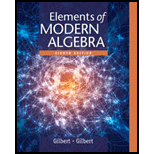# a. Give an example where a and b are not zero divisors in a ring R , but the sum a + b is a zero divisor. Give an example where a and b are zero divisors in a ring R with a + b ≠ 0 , and a + b is not a zero divisor. Prove that the set of all elements in a ring R that are not zero divisors is closed under multiplication.### Elements Of Modern Algebra

8th Edition
Gilbert + 2 others
Publisher: Cengage Learning,
ISBN: 9781285463230

#### Solutions

Chapter
Section### Elements Of Modern Algebra

8th Edition
Gilbert + 2 others
Publisher: Cengage Learning,
ISBN: 9781285463230
Chapter 5.2, Problem 19E
Textbook Problem
43 views

## a. Give an example where a and b are not zero divisors in a ring R , but the sum a   +   b is a zero divisor.Give an example where a and b are zero divisors in a ring R with a   +   b   ≠   0 , and a   +   b is not a zero divisor.Prove that the set of all elements in a ring R that are not zero divisors is closed under multiplication.

(a)

To determine

An example where a and b are not zero divisors in a ring R, but the sum a+b is a zero divisor.

### Explanation of Solution

Let R=8={,,,,,,,} be a ring.

For  and  in 8, there doesn’t exist any [a] element in 8.

Such that [a]= or [a]=.

 and  are not zero divisors in a ring 8

(b)

To determine

An example where a and b are zero divisors in a ring R with a+b0, and a+b is not a zero divisor.

(c)

To determine

To prove: The set of all elements in a ring, R that are not zero divisors, is closed under multiplication.

### Still sussing out bartleby?

Check out a sample textbook solution.

See a sample solution

#### The Solution to Your Study Problems

Bartleby provides explanations to thousands of textbook problems written by our experts, many with advanced degrees!

Get Started

Find more solutions based on key concepts
Find the differential of each function. 14. (a) y = ln(sin ) (b) y=ex1ex

Single Variable Calculus: Early Transcendentals, Volume I

Convert 50 miles per hour to feet per second.

Trigonometry (MindTap Course List)

In Exercises 39-50, sketch the graph of the function with the given rule. Find the domain and range of the func...

Applied Calculus for the Managerial, Life, and Social Sciences: A Brief Approach

Is it possible to obtain a negative value for the variance or the standard deviation?

Statistics for The Behavioral Sciences (MindTap Course List)

In Exercises 3336, find an equation of line that has slope m and y-intercept b. m=12; b=34

Finite Mathematics for the Managerial, Life, and Social Sciences

If 13f(x)dx=10 and 13g(x)dx=6, then 13(2f(x)3g(x))dx= a) 2 b) 4 c) 18 d) 38

Study Guide for Stewart's Single Variable Calculus: Early Transcendentals, 8th

True or False: For f(x, y) = x2(x + y), x and y are independent variables.

Study Guide for Stewart's Multivariable Calculus, 8th